## Chopper Controlled Resistance:

By interrupting the flow of current through a resistance R in a periodic fashion using a switch, the value of resistance can be effectively varied. The interrup­tion of the current can be accomplished by means of a dc chopper connected across the resistance, working as a switching device. When the chopper is on, the resistance is short circuited and the current is diverted through the Chopper Controlled Resistance. When it is OFF, the supply voltage is connected to the resistance and the current flows through the resistance. If the chopper is always ON the effective resistance is zero. The effective resistance is ft if the chopper is always OFF. Thus by controlling the ON/OFF ratio of the chopper, the effec­tive resistance can he controlled in the range 0-R. Under ideal conditions the effective resistance can be shown to be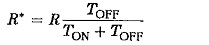The chopper controlling the resistance is depicted in Fig. 3.89(a). The fig­ure also gives the current waveform through the resistance. The voltage is in the form of pulses and the resistance is also called a pulse controlled resis­tance. The smoothing inductance L is used to reduce the ripple in the load current.Sometimes a correction for the simple expression above may be required if the R-C time constant is not negligible compared to the chopper period T. In this case the capacitor current flowing through the resistance alters its effective value. Taking this into consideration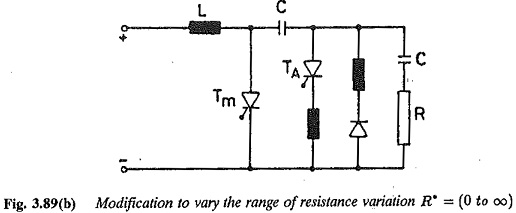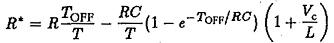The resistance can be varied from 0 to ∞ by a simple modification in the circuit, as shown in Fig. 3.89(b). A condenser is connected in series with the resistance and an R-C combination is placed across the chopper. When the chopper is always OFF, the capacitor does not allow any current through the resistance and the value of effective resistance is ∞. However, there is now a danger of high voltage across the main thyristor.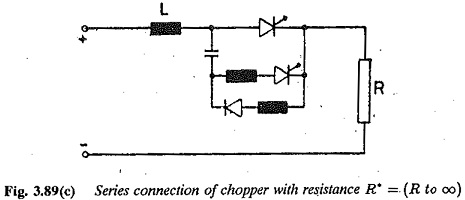The chopper can also be connected in series with the resistance, as shown in Fig. 3.89(b). When the chopper is ON the resistance is R and when it is OFF it is infinity. By varying TON/TOFF, the resistance can be varied from ft to infinity. In this case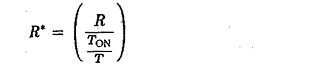TON/T can be varied from 1 to 0 and R* varies from R to ∞.

Scroll to Top